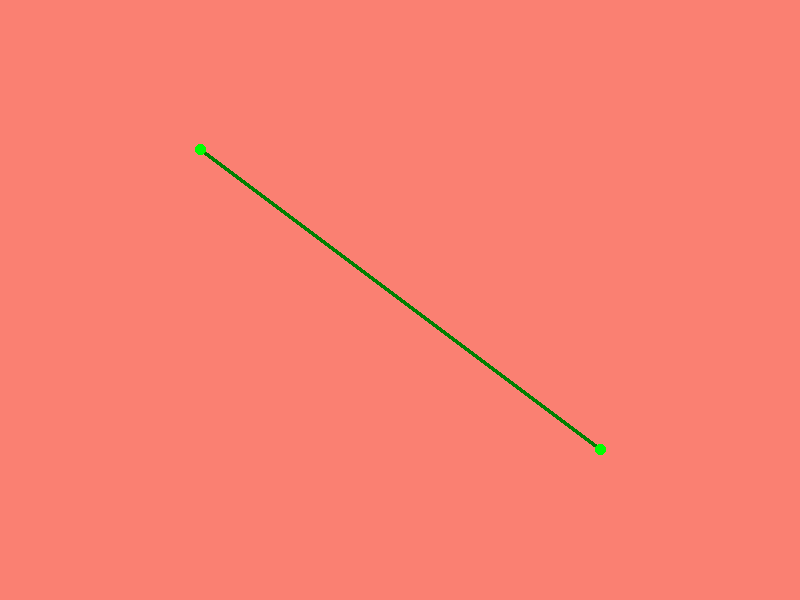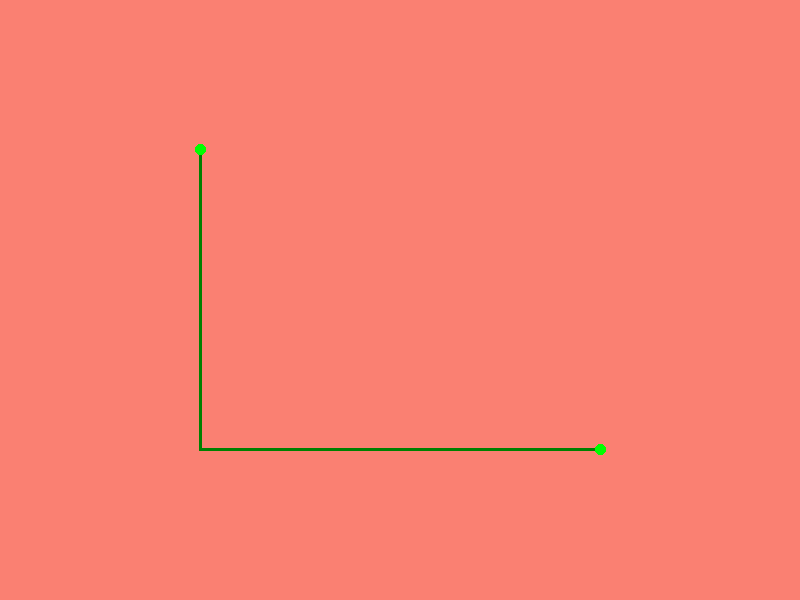# Pathfinding#

## Importing Packages#

:

import numpy as np

import xrspatial


## A*#

A* is an informed search algorithm, or a best-first search, meaning that it is formulated in terms of weighted graphs: starting from a specific starting node of a graph, it aims to find a path to the given goal node having the smallest cost (min distance travelled, shortest time, …).

The xrspatial.a_star_search function calculate the shortest path in pixel space from a start location to a goal location through a given aggregate surface graph. The graph should be a line raster which contains crossable and non-crossable (a.k.a walls or barrieres) values. Note that both start and goal are in (lon, lat), or (x, y) coordinate space and must be within the graph. The xrspatial.a_star_search provides 2 separate options, snap_start and snap_goal, which can be set true to snap locations to the nearest valid value before beginning pathfinding. It also provides connectivity option to indicate neighborhood structure. This value can be set to either 4 or 8 that represents for 4-connectivity and 8-connectivity accordingly.

Let’s generate some fake line raster and find shortest path with A*.

:

from xrspatial import a_star_search
import pandas as pd

# define range of x and y
xrange = (0, 4)
yrange = (0, 4)

# create line raster
ys = [1, 1, 3, 3, 1, 1, np.nan, 1, 3, np.nan, 1, 3, np.nan, 1, 3, np.nan, 2, 2]
xs = [1, 3, 3, 1, 1, 3, np.nan, 1, 3, np.nan, 3, 1, np.nan, 2, 2, np.nan, 1, 3]
line_df = pd.DataFrame(dict(x=xs, y=ys))

W = 800
H = 600
cvs = ds.Canvas(plot_width=W, plot_height=H,
x_range=xrange, y_range=yrange)
line_agg = cvs.line(line_df, x='x', y='y').astype(int)

# pick up 2 random locations
start = (1, 3)
goal = (3, 1)

location_df = pd.DataFrame({'x': [start, goal], 'y': [start, goal]})
location_agg = cvs.points(location_df, 'x', 'y')


:### 8-connectivity#

:

# find the path from start to goal,
# barriers are uncrossable cells. In this case, they are cells with a value of 0

path_agg_8_connectivity = a_star_search(line_agg, start, goal, barriers=, snap_start=True, snap_goal=True)


:### 4-connectivity#

:

# find the path from start to goal,
# barriers are uncrossable cells. In this case, they are cells with a value of 0

path_agg_4_connectivity = a_star_search(line_agg, start, goal, barriers=,
snap_start=True, snap_goal=True, connectivity=4)


: